# While Loops in Java

## Description

In computer programming, a loop is a sequence of instruction s that is continually repeated until a certain condition is reached. Imagine you have to write a program which performs a repetitive task such as printing 1 to 100. Writing 100 print statements would not be wise thing to do. Loops are specifically designed to perform repetitive tasks with one set of code. Loops save a lot of time. A loop is a structure that allows repeated execution of a block of statements. Within a looping structure, a Boolean expression is evaluated. If it is true, a block of statements called the loop body executes and the Boolean expression is evaluated again. As long as the expression is true, the statements in the loop body continue to execute. When the Boolean evaluation is false, the loop ends.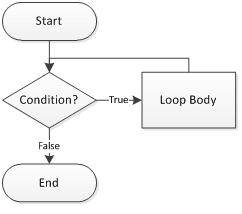In Java there are three types of loops:

• A while loop, in which the loop-controlling Boolean expression is the first statement in the loop
• A for loop, which is usually used as a concise format in which to execute loops
• A do...while loop, in which the loop-controlling Boolean expression is the last statement in the loop

While Loops

The while loop is good for scenarios where you don't know how many times a block or statement should repeat, but you want to continue looping as long as some condition is true. A while statement looks like below. In Java, a while loop consists of the keyword while followed by a Boolean expression within parentheses, followed by the body of the loop, which can be a single statement or a block of statements surrounded by curly braces.

```while (expression) {// do stuff}
```

You can use a while loop when you need to perform a task a predetermined number of times. A loop that executes a specific number of times is a definite loop or a counted loop. To write a definite loop, you initialize a loop control variable, a variable whose value determines whether loop execution continues. While the Boolean value that results from comparing the loop control variable and another value is true, the body of the while loop continues to execute. In the body of the loop, you must include a statement that alters the loop control variable.

Java Code: Go to the editor

``````public class WhileLoopDemo {
public static void main(String[] args) {
int var = 1;
int limit=11;
while (var < limit)
{
System.out.println("Loop counter: " + var);
var++;
}
}
}
```
```

Output: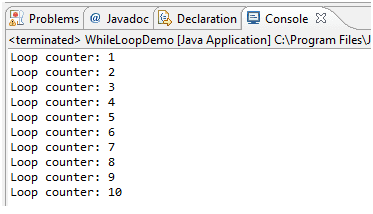The key point to remember about a while loop is that it might not ever run. If the test expression is false the first time the while expression is checked, the loop body will be skipped and the program will begin executing at the first statement after the while loop.Let’s take an example to understand this. In below program user enters the value of loop counter variable, If counter variable is less than 5, then the loop will execute and increment the counter variable by one till counter value is equal to 5. If counter variable is more than or equal to 5, the loop will not execute.

Java Code: Go to the editor

``````import java.util.Scanner;
public class WhileLoopNegativeCondition {
public static void main(String[] args)
{
int counter;
Scanner inputDevice = new Scanner(System.in);
System.out.print("Please enter loop counter value >> ");
counter = inputDevice.nextInt();
System.out.println("Before Loop");
while (counter < 5)
{
System.out.println("Inside Loop- Counter= "+ counter);
counter++;
}
System.out.println("After While Loop");
}
}
```
```

Output: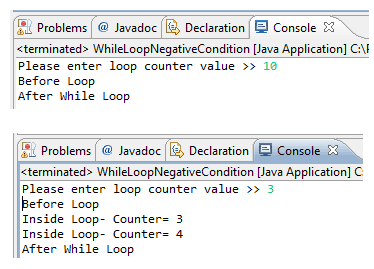Important Points while using loops

• Loop condition/expression can be true always, which makes our loop infinite. This is bad programming practice as it might result in memory exception. Below statement is valid but not good to have in our program.
```while (true) {// Some stuff
```
```while (2<4)// Some stuff
```
• It is very common to alter the value of a loop control variable by adding 1 to it or incrementing the variable. However, not all loops are controlled by adding 1 sometimes we can control by subtracting 1 from a loop control variable or decrementing it.

do … while Loops

The do loop is similar to the while loop, except that the expression is not evaluated until after the do loop's code is executed. Therefore the code in a do loop is guaranteed to execute at least once. The following shows a do loop syntax:

``` do {//Loop Body} while(Condition);
```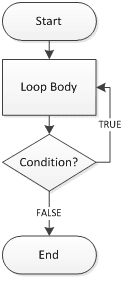If so, you want to write a loop that checks at the “bottom” of the loop after the first iteration. The do...while loop checks the value of the loop control variable at the bottom of the loop after one repetition has occurred. Below sample code explain do… while loop.

Java Code: Go to the editor

``````public class DoWhileLoopDemo {
public static void main(String[] args) {
int i =10;
do{
i=i+10;
System.out.println("Loop Counter Variable=" +i);
}
while (i< 10);
}
}
```
```

Output: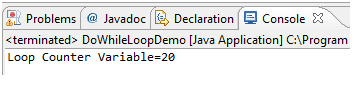Summary

• The while loop is used for scenarios where you don't know how many times a block of code should be executed.
• The do loop is similar to the while loop, except that the expression is not evaluated until after the do loop's code is executed.

Java Code Editor:

Previous: Switch Satement
Next: For loop

﻿